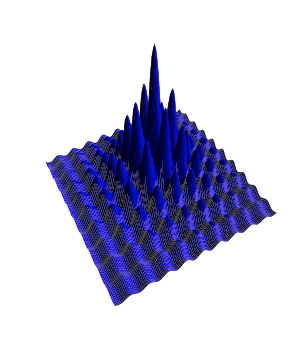Want to share your content on R-bloggers? click here if you have a blog, or here if you don't.

I have been working on a reliable optimization method for this crazy function.

```f.egg<-function(x,y){
(2+cos(x)+cos(y))/(100+x^2+y^2)
}

```I noticed that if I had a large variance in the random normal generator, the optimizer would jump all over the place but would not settle down in the best optimum. So I added in a second step that takes the best of the attempted values and uses them as a second set of start values. Then, a second smaller variance is used that does not allow for major jumps. The two step anneal function can be seen below.

```twostep.anneal<-function(f,mu,n=1000,sig=c(1,.1),t=1,g=0.999){
xm = mu
ym = mu
fm = f(xm,ym)
x = rep(NA,n*2)
y = rep(NA,n*2)
fx = rep(NA,n*2)
for(k in 1:2){
s=sig[k]
if(k==2){
xm=best
ym=best
}
for (i in 1:n){
dxm = xm+rnorm(1,0,s)
dym = ym+rnorm(1,0,s)
fdm = f(dxm, dym)

t = t*g
if (runif(1) < (fdm/fm)^(1/t)){
xm = dxm
ym = dym
fm = fdm
}
x[(i+(k-1)*n)] = xm
y[(i+(k-1)*n)] = ym
fx[(i+(k-1)*n)] = fm
}
ii = which(fx==max(fx, na.rm=TRUE))
best=c(x[ii], y[ii])
}
list(x = x, y = y, fx = fx, best = best , fbest = fx[ii], t=t)
}

```

To run the function and see the path of the anneal function use this code.

```x<-seq(-30,30,.5)
y<-seq(-30,30,.5)

z<-outer(x,y, f.egg)

aa<-twostep.anneal(f.egg, n=1000, sig=c(2, 2), mu=c(5,5), t=2, g=.99)

contour(x,y,z)
lines(aa\$x, aa\$y, col=2)
```